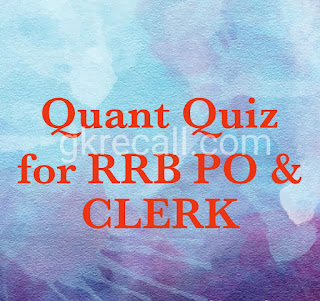# gkrecall

We brings you daily Current affairs, daily Current Affairs Quiz, weekly Current Affairs, weekly Current Affairs Quiz to enhance your preparation for upcoming exams. We also provide articles related to quant, reasoning, English, ssc etc .

##RRB Clerk and RRB PO Prelims exam are going to be held in the upcoming months. GKRECALL have already provided you with the PDFs of many topics of Quantitative Aptitude like Simplification/Approximation, NumberSeries,datainterpretation.important .
Arithmetic questions to prepare for RRB Clerk and PO Pre exam. Practicing these questions will help you to know about the level of the questions. To increase your speed and accuracy, enhance your calculations.

Question 1
The marked price of a Timex sports watch was Rs. 1440. A man bought the same for Rs. 864 after getting two successive discounts, the first being 20%. What was the second discount rate?

A12%
B14%
C15%
D18%
ENone of these

Question 2
A Raymond Showroom keeper marked the price of his commodity so as to include a profit of 40%. He allowed discount of 10% on the marked price. His actual profit was.

A12%
B26%
C15%
D18%
ENone of these

The price of a Laughing Buddha Statue is raised by 50% and then two successive discounts of 15% each are allowed. Ultimately, the price of the Statue is

A.decreased by 8.375%
B.increased by 3%
C.increased by 8.375%
D.increased by 10%
E.None of these

Question 4
A shopkeeper sells a YONEX badminton racket, whose marked price is Rs. 100, at a discount of 20% and gives a shuttle cock costing Rs. 10 free with each racket. Even then he makes a profit of 20%. His cost price per racket is

ARs. 69.75
BRs. 65
CRs. 70
DRs. 58.33
ENone of these

Question 5
A shopkeeper sold Banarasi sarees at Rs. 361 each after giving 5% discount on labeled price. Had he not given the discount, he would have earned a profit of 52% on the cost price. What was the cost price of each Banarasi saree?

ARs. 240
BRs. 260
CRs. 280
DNone of these
E300

Question 6
A man buys an article for 15% less than its value and sells it for 15% more than its value. His gain or loss percent is:

Ano profit, no loss
B20% profit
Cless than 20% profit
Dmore than 20% profit
ENone of these

Question 7
A dealer marked up his goods 20% above its cost price and sold 80% of the goods at 25% gain and remaining at marked price. Find his overall gain percentage.

A25%
B24%
C26%
D30%
E22%

Question 8
Tarun invested Rs X in scheme I for three years at the rate of 12% p.a. at simple interest while Khushi invested Rs (X + 4000) in scheme II for 2 years on C.I. at 20% p.a. rate. Find sum invested by Khushi if total interest earned by them is Rs. 3360.

A4400
B4500
C4000
D4600
ENone of these

Question 9
A milkman made a mixture by mixing milk and water in the ratio 2 : 3 and sold 37.5% of the mixture. If he added 62.5 ℓ water in the remaining mixture, ratio of milk to water becomes 2 : 5, then Find the initial quantity of mixture?

A250 ℓ
B200 ℓ
C450 ℓ
D350 ℓ
E500 ℓ

Question 10
CI on a certain sum for 2 years is Rs. 80.80 and SI is Rs. 80 for same years. What will be the rate of interest?

A.2%
B. 4%
C.6%
D.5%
E.8%

SOLUTIONS:

1.let the 2nd discount be Rs.x.
ATQ,

x = 25%

2.Let CP = 100,MP=140,SP = 126
Actual profit = 126 - 100 = 26
% profit = 26/100 × 100 = 26%

3.Let the CP=100,MP=150
ATQ,150 × 85/100× 85/100=108.375
% profit = 8.375%

4.MP = 100
SP = 80 - 10 = 70
CP=70 × 100/120= 58.33

5.SP = 361,MP = 361×100/95= 380
CP = 380 ×100/152  = 250

6.Let actual cost price be Rs.100
Cost price of man =100 ×85/100= 85
SP of man=100 × 115/100= 115
% Profit =30/85 ×100 = 35 5/17%

7.Let CP of on item be = Rs.100
Total quantity of item = 100
Then total CP = 100 × 100 = 10000
SP of items which sold at 25% gain = 80 ×125 = 10000
SP of items which sold at MP =20 × 120 = 2400
Profit percentage =
2400/10000  × 100 = 24%

8.Let amount invested in scheme be = x
ATQ,

x=2000
Amount invested by Khushi = 2000 + 4000 = 6000

9.As given in the question 37.5%=3/8
part is sold.so remaining part is =5/8

x = 50
So initial quantity of mixture 5 × 50 = 250 ℓ